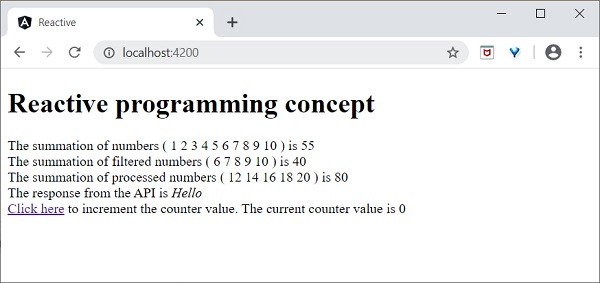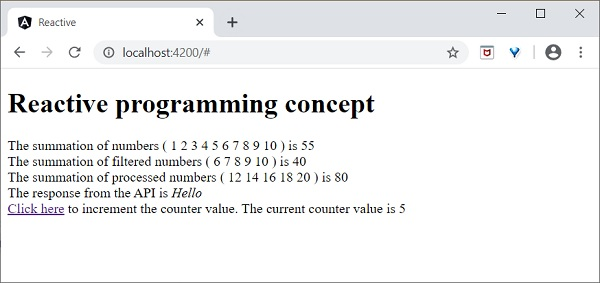# Angular 8 - Reactive Programming

Reactive programming is a programming paradigm dealing with data streams and the propagation of changes. Data streams may be static or dynamic. An example of static data stream is an array or collection of data. It will have an initial quantity and it will not change. An example for dynamic data stream is event emitters. Event emitters emit the data whenever the event happens. Initially, there may be no events but as the time moves on, events happens and it will gets emitted.

Reactive programming enables the data stream to be emitted from one source called Observable and the emitted data stream to be caught by other sources called Observer through a process called subscription. This Observable / Observer pattern or simple Observer pattern greatly simplifies complex change detection and necessary updating in the context of the programming.

JavaScript does not have the built-in support for Reactive Programming. RxJs is a JavaScript Library which enables reactive programming in JavaScript. Angular uses RxJs library extensively to do below mentioned advanced concepts −

• Data transfer between components.
• HTTP client.
• Router.
• Reactive forms.

Let us learn reactive programming using RxJs library in this chapter.

## Observable

As learn earlier, Observable are data sources and they may be static or dynamic. Rxjs provides lot of method to create Observable from common JavaScript Objects. Let us see some of the common methods.

of − Emit any number of values in a sequence and finally emit a complete notification.

const numbers$= of(1, 2, 3, 4, 5, 6, 7, 8, 9, 10);  Here, • numbers$ is an Observable object, which when subscribed will emit 1 to 10 in a sequence.

• Dollar sign ($) at the end of the variable is to identify that the variable is Observable. range − Emit a range of number in sequence. const numbers$ = range(1,10)


from − Emit array, promise or iterable.

const numbers$= from([1,2,3,4,5,6,7,8,9,10]);  ajax − Fetch a url through AJAX and then emit the response. const api$ = ajax({ url: 'https://httpbin.org/delay/1', method: 'POST', headers: { 'Content-Type': 'application/text' }, body: "Hello" });


Here,

https://httpbin.org is a free REST API service which will return the supplied body content in the JSON format as specified below −

{
"args": {},
"data": "Hello",
"files": {},
"form": {},
"Accept": "text/html,application/xhtml+xml,application/xml;q=0.9,image/webp,image/apng,*/*;q=0.8,application/signed-exchange;v=b3;q=0.9",
"Accept-Encoding": "gzip, deflate, br",
"Accept-Language": "en-US,en;q=0.9",
"Host": "httpbin.org", "Sec-Fetch-Dest": "document",
"Sec-Fetch-Mode": "navigate",
"Sec-Fetch-Site": "none",
"User-Agent": "Mozilla/5.0 (Windows NT 10.0; Win64; x64) AppleWebKit/537.36 (KHTML, like Gecko) Chrome/83.0.4103.106 Safari/537.36",
"X-Amzn-Trace-Id": "Root=1-5eeef468-015d8f0c228367109234953c"
},
"url": "https://httpbin.org/delay/1"
}


fromEvent − Listen to an HTML element’s event and then emit the event and its property whenever the listened event fires.

const clickEvent$= fromEvent(document.getElementById('counter'), 'click');  Angular internally uses the concept extensively to provide data transfer between components and for reactive forms. ## Subscribing process Subscribing to an Observable is quite easy. Every Observable object will have a method, subscribe for the subscription process. Observer need to implement three callback function to subscribe to the Observable object. They are as follows − • next − Receive and process the value emitted from the Observable • error − Error handling callback • complete − Callback function called when all data from Observable are emitted. Once the three callback functions are defined, Observable’s subscribe method has to be called as specified below − const numbers$ = from([1,2,3,4,5,6,7,8,9,10]);
// observer
const observer = {
next: (num: number) => {      this.numbers.push(num); this.val1 += num },
error: (err: any) => console.log(err),
complete: () => console.log("Observation completed")
};
numbers$.subscribe(observer);  Here, • next − method get the emitted number and then push it into the local variable, this.numbers. • next − method also adding the number to local variable, this.val1. • error − method just writes the error message to console. • complete − method also writes the completion message to console. We can skip error and complete method and write only the next method as shown below − number$.subscribe((num: number) => { this.numbers.push(num); this.val1 += num; });


## Operations

Rxjs library provides some of the operators to process the data stream. Some of the important operators are as follows −

filter − Enable to filter the data stream using callback function.

const filterFn = filter( (num : number) => num > 5 );
const filteredNumbers$= filterFn(numbers$);
filteredNumbers$.subscribe( (num : number) => { this.filteredNumbers.push(num); this.val2 += num } );  map − Enables to map the data stream using callback function and to change the data stream itself. const mapFn = map( (num : number) => num + num ); const mappedNumbers$ = mappedFn(numbers$);  pipe − Enable two or more operators to be combined. const filterFn = filter( (num : number) => num > 5 ); const mapFn = map( (num : number) => num + num ); const processedNumbers$ = numbers$.pipe(filterFn, mapFn); processedNumbers$.subscribe( (num : number) => { this.processedNumbers.push(num); this.val3 += num } );


Let us create a sample application to try out the reaction programming concept learned in this chapter.

Create a new application, reactive using below command −

ng new reactive


Change the directory to our newly created application.

cd reactive


Run the application.

ng serve


Change the AppComponent component code (src/app/app.component.ts) as specified below −

import { Component, OnInit } from '@angular/core'; import { Observable, of, range, from, fromEvent } from 'rxjs';
import { ajax } from 'rxjs/ajax';
import { filter, map, catchError } from 'rxjs/operators';
@Component({
selector: 'app-root',
templateUrl: './app.component.html',
styleUrls: ['./app.component.css']
})
export class AppComponent implements OnInit {
title = 'Reactive programming concept';
numbers : number[] = [];
val1 : number = 0;
filteredNumbers : number[] = [];
val2 : number = 0;
processedNumbers : number[] = [];
val3 : number = 0;
apiMessage : string;
counter : number = 0;
ngOnInit() {
// Observable stream of data Observable<number>
// const numbers$= of(1, 2, 3, 4, 5, 6, 7, 8, 9, 10); // const numbers$ = range(1,10);
const numbers$= from([1,2,3,4,5,6,7,8,9,10]); // observer const observer = { next: (num: number) => {this.numbers.push(num); this.val1 += num }, error: (err: any) => console.log(err), complete: () => console.log("Observation completed") }; numbers$.subscribe(observer);
const filterFn = filter( (num : number) => num > 5 );
const filteredNumbers = filterFn(numbers$); filteredNumbers.subscribe( (num : number) => {this.filteredNumbers.push(num); this.val2 += num } ); const mapFn = map( (num : number) => num + num ); const processedNumbers$ = numbers$.pipe(filterFn, mapFn); processedNumbers$.subscribe( (num : number) => {this.processedNumbers.push(num); this.val3 += num } );
const api$= ajax({ url: 'https://httpbin.org/delay/1', method: 'POST', headers: {'Content-Type': 'application/text' }, body: "Hello" }); api$.subscribe(res => this.apiMessage = res.response.data );
const clickEvent$= fromEvent(document.getElementById('counter'), 'click'); clickEvent$.subscribe( () => this.counter++ );
}
}


Here,

• Used of, range, from, ajax and fromEvent methods to created Observable.
• Used filter, map and pipe operator methods to process the data stream.
• Callback functions catch the emitted data, process it and then store it in component’s local variables.

Change the AppComponent template (src/app/app.component.html) as specified below −

<h1>{{ title }}</h1>
<div>
The summation of numbers ( <span *ngFor="let num of numbers"> {{ num }} </span> ) is {{ val1 }}
</div>
<div>
The summation of filtered numbers ( <span *ngFor="let num of filteredNumbers"> {{ num }} </span> ) is {{ val2 }}
</div>
<div>
The summation of processed numbers ( <span *ngFor="let num of processedNumbers"> {{ num }} </span> ) is {{ val3 }}
</div>
<div>
The response from the API is <em>{{ apiMessage }}</em> </div>
<div>
<a id="counter" href="#">Click here</a> to increment the counter value. The current counter value is {{ counter }}
<div>


Here,

Shown all the local variable processed by Observer callback functions.

Open the browser, http://localhost:4200.Click the Click here link for five times. For each event, the event will be emitted and forward to the Observer. Observer callback function will be called. The callback function increment the counter for every click and the final result will be as shown below −